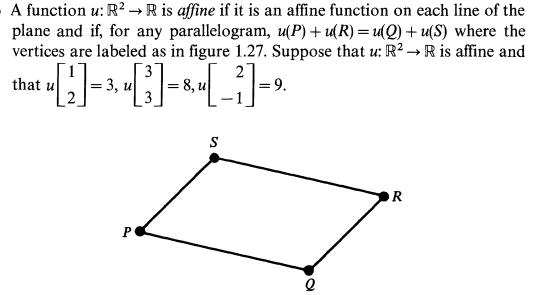# Find the formula for an affine function

• Aleoa

## Homework Statement## The Attempt at a Solution

I don't understand what an affine function $\mathbb{R}^{2}\rightarrow \mathbb{R}$ expresses... I don't know if it's a coordinate function.

And i also don't understand (i'm sorry) why for any parallelogram, u(P) + u(R) = u(Q) + u(S)

#### Attachments

I don't understand what an affine function $\mathbb{R}^{2}\rightarrow \mathbb{R}$ expresses...
It's a function of the form ##\pmatrix{x\\y}\mapsto ax+by+c## for some real constants ##a,b,c##.
And i also don't understand (i'm sorry) why for any parallelogram, u(P) + u(R) = u(Q) + u(S)
This may be easier to understand if you deduct (u(R)+u(Q)) from both sides and then think of it in terms of vectors that are edges of the parallelogram.

An affine space is one in which the difference of any two elements is a vector, so it's usually easier to work with things in an affine space when they are expressed in terms of the difference of two elements. Performing the operation described in the previous paragraph does just that.

•Aleoa
It's a function of the form ##\pmatrix{x\\y}\mapsto ax+by+c## for some real constants ##a,b,c##.

This may be easier to understand if you deduct (u(R)+u(Q)) from both sides and then think of it in terms of vectors that are edges of the parallelogram.

An affine space is one in which the difference of any two elements is a vector, so it's usually easier to work with things in an affine space when they are expressed in terms of the difference of two elements. Performing the operation described in the previous paragraph does just that.

Thanks so much. The formula is F=3x-y+2.

And, the lines u = constant in R^2 are all the lines perpendicular to the vector [1,3]. Is it correct ?

the lines u = constant in R^2 are all the lines perpendicular to the vector [1,3]. Is it correct ?
Perpendicular? or Parallel? Check which it is by substituting in a couple of values on the line y=3x, then a couple on a line perpendicular to that, and see for which pair the function gives the same value.

•Aleoa